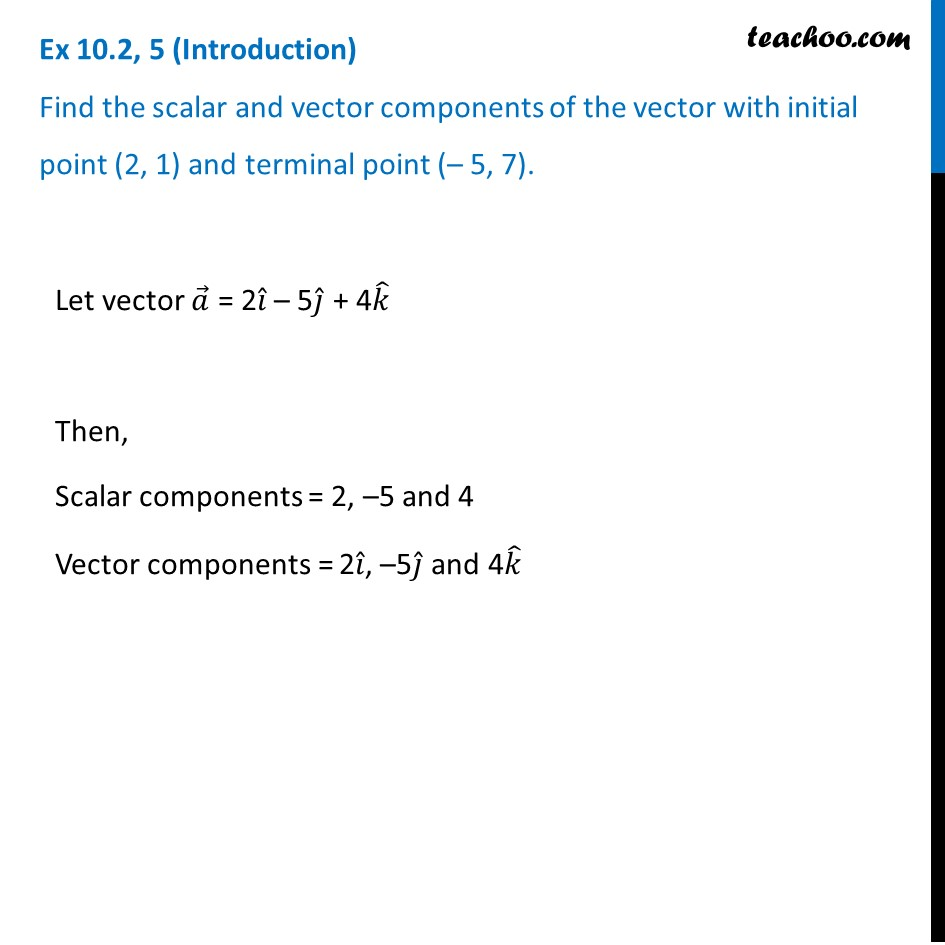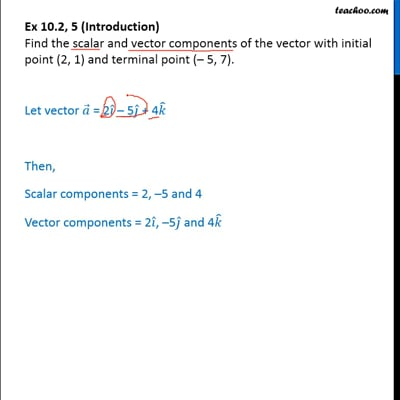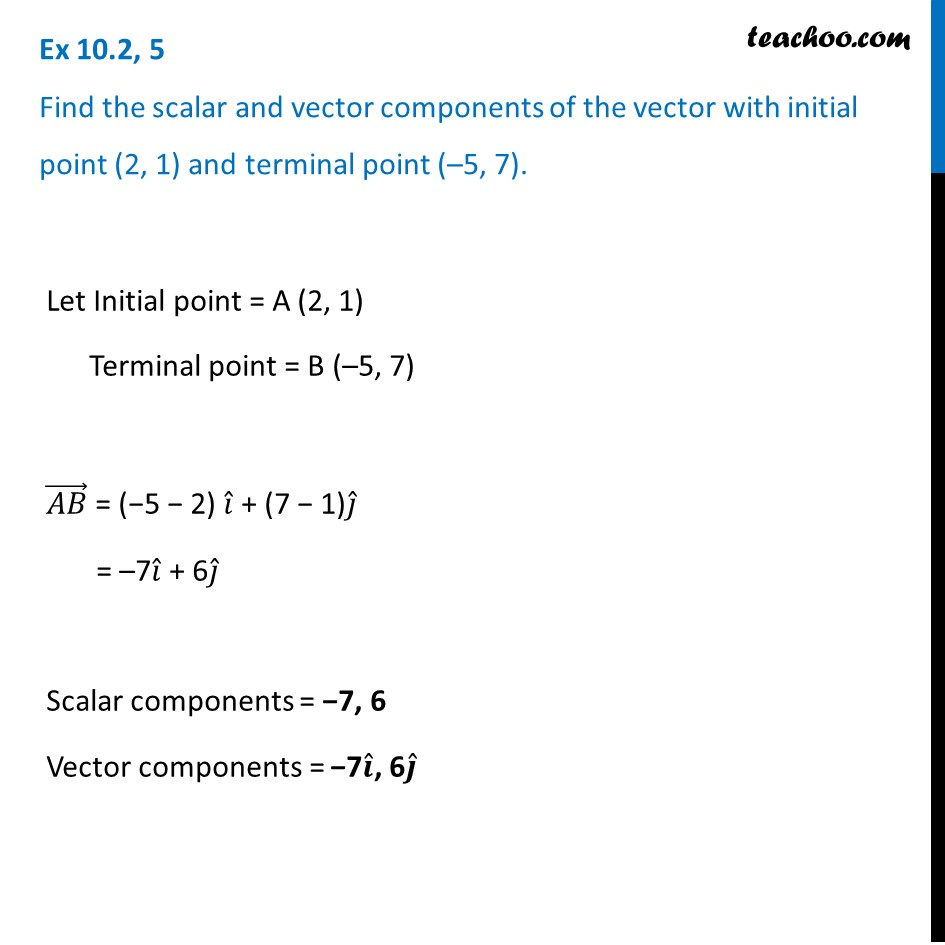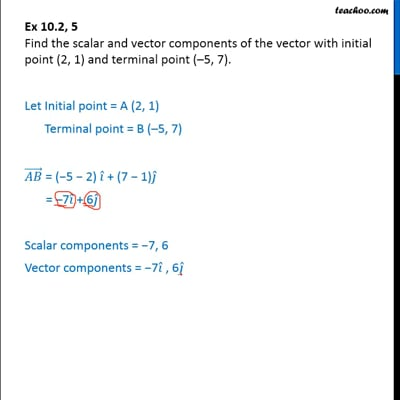Joining two points

Chapter 10 Class 12 Vector Algebra
Concept wiseThis video is only available for Teachoo black usersThis video is only available for Teachoo black users

Introducing your new favourite teacher - Teachoo Black, at only ₹83 per month

### Transcript

Ex 10.2, 5 (Introduction) Find the scalar and vector components of the vector with initial point (2, 1) and terminal point (– 5, 7). Let vector 𝑎 ⃗ = 2𝑖 ̂ – 5𝑗 ̂ + 4𝑘 ̂ Then, Scalar components = 2, –5 and 4 Vector components = 2𝑖 ̂, –5𝑗 ̂ and 4𝑘 ̂ Ex 10.2, 5 Find the scalar and vector components of the vector with initial point (2, 1) and terminal point (–5, 7). Let Initial point = A (2, 1) Terminal point = B (–5, 7) (𝐴𝐵) ⃗ = (−5 − 2) 𝑖 ̂ + (7 − 1)𝑗 ̂ = –7𝑖 ̂ + 6𝑗 ̂ Scalar components = −7, 6 Vector components = −7𝒊 ̂, 6𝒋 ̂